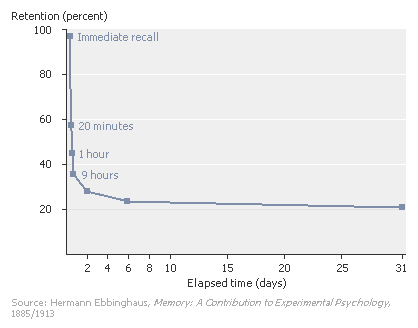Search IntMath
Close

450+ Math Lessons written by Math Professors and Teachers

5 Million+ Students Helped Each Year

1200+ Articles Written by Math Educators and Enthusiasts

Simplifying and Teaching Math for Over 23 Years

# IntMath Newsletter - Overcoming fear of math tests, free calculus book

By Murray Bourne, 26 Apr 2009

1. Math Tip - Overcoming fear of math tests
2. Math Tip - An excellent free calculus text book
3. The forgetting curve
4. From the Math Blog
5. Final Thought - Thinking you can

## 1. Math Tip - Overcoming fear of math tests

In last month's IntMath Poll, readers indicated that they fear math tests more than all the usual phobias (spiders, heights and so on).

I promised to write an article with tips on how you can reduce your fear of math tests, so here it is:

Overcoming your fear of math tests

## 2. Math Tip - An excellent free calculus text book

Most students have trouble understanding calculus concepts.

I came across a great book that does an excellent job of explaining what is really going on in calculus. This is a good book for students (it will give you a better understanding about calculus) and teachers (since it will help you to explain calculus better.)

See the article and get the book (in PDF form) here:

## 3. The forgetting curve

Did you know that forgetting is an important part of learning?

We cannot possibly remember everything we read, see, hear or experience, so our brains need to constantly make a decision — is this piece of information vital for my future? If yes, then a weak memory is formed. If no, it is discarded to make way for more important or interesting things.

Around 100 years ago, philosopher Hermann Ebbinghaus conducted experiments on memory. He memorized a set of 3-letter nonsense syllables and then tested himself at intervals to see how much he could remember. The result was as follows:[Source: Encarta (link no longer available)]

Within one day, he had forgotten more than half of what he had learned. Of course, there was no meaning in what he was learning and clearly, if you understand what you are learning and it has significance for you, then you will remember it better than the above curve indicates. But if you take a "surface learning" approach, it is very difficult to remember those facts later.

There are many implications in this. If your math lesson is meaningless (you don't understand it, or you weren't listening), then anything you picked up will be gone from your memory within a few days. But if you go home that night and work on that math lesson (do the homework, practice the graph drawing, learn the formulas), there is a far better chance that you will retain it for longer and the next math lesson will make much more sense.

Before I leave this topic I want to point out some interesting math behind that forgetting curve above. It is very close to an exponential decay curve, which you can read about here: Graphs of Exponential and Logarithmic Curves.

We could model the above curve using the following exponential decay equation:

R = et/s,

where R = retention, t = time and s = relative strength of the memory.

Of course, if the memory is weak to start with, it will fade quickly. Practice and processing helps to form a stronger memory, which will be retained longer.

## 4. From the Math Blog

I mentioned the first 2 of these earlier in this Newsletter.

A) Overcoming your fear of math tests
Some people seem very calm as they head into a math test. What's their secret?

B) Calculus Made Easy (Free book)
Here's a great introductory book for calculus — and it's free!

C) Mathematics Carnival 51
A collection of interesting posts from the math blogosphere.

D) Math of plastic water bottles
Plastic water bottles are a disaster for the environment — and your wallet.

E) Chocolate math
Sharing chocolate with your spouse is not always a fair affair.

F) Friday math movie - The Miniature Earth
What if the world only had 100 people? What would characterize such a world?

## 5. Final Thought - Thinking you can

To finish this edition of the IntMath Newsletter, here's a great quote from Henry Ford:

Whether you think you can or whether you think you can't, you're right.

Until next time, enjoy whatever you learn.

### 7 Comments on “IntMath Newsletter - Overcoming fear of math tests, free calculus book”

1. waqaraziz says:

sir,
send me some thng about differential equations.
Thanks

2. Murray says:

Hi waqaraziz. You can find the chapter on DEs here: Differential Equations.

Good luck with it!

3. Magdy W. S. says:

Thanks very much! I do enjoy reading these articles.

4. Johann (Lemmie) van der Linde says:

As always, no comment but just a very big thank you for your letter. If possible more e-books please; the fun stuff.(coffee table material) that you can put down and get people hooked.

Regards.
Lemmie

5. Saw Aung Kyaw Oo says:

Sir,

Thanks again, especially for the book " Calculus made easy".
Are math tutor dvds available in S'pore?

Regards
SAW

6. Murray says:

Hi Saw. You're welcome!

The DVDs are only available from the developer in the USA: MathTutorDVD.

7. Lakshman says:

Very good one I can say
It really helps.........

### Comment Preview

HTML: You can use simple tags like <b>, <a href="...">, etc.

To enter math, you can can either:

1. Use simple calculator-like input in the following format (surround your math in backticks, or qq on tablet or phone):
a^2 = sqrt(b^2 + c^2)
(See more on ASCIIMath syntax); or
2. Use simple LaTeX in the following format. Surround your math with $$ and $$.
$$\int g dx = \sqrt{\frac{a}{b}}$$
(This is standard simple LaTeX.)

NOTE: You can mix both types of math entry in your comment.